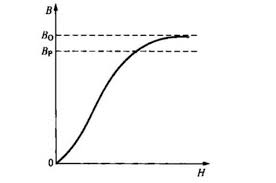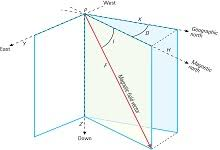## How to Calculate and Solve for Intensity of Induced Magnetization (K, H) | Magnetic FieldThe image above represents intensity of induced magnetization.

To compute for intensity of induced magnetization, two essential parameters are needed and these parameters are Magnetic Susceptibility (K) and Magnetizing Force of Inducing Field (H).

The formula for calculating intensity induced magnetization:

J = KH

Where:

J = Intensity of Induced Magnetization
K = Magnetic Susceptibility
H = Magnetizing Force of Inducing Field

Let’s solve an example;
Find the intensity induced magnetization when the magnetic susceptibility is 14 and the magnetizing force of inducing field is 24.

This implies that;

K = Magnetic Susceptibility = 14
H = Magnetizing Force of Inducing Field = 24

J = KH
J = (14)(24)
J = 336

Therefore, the intensity induced magnetization is 336.

Calculating the Magnetic Susceptibility when the Intensity Induced Magnetization and the Magnetizing Force of Induced Field is Given.

K = J / H

Where;

K = Magnetic Susceptibility
J = Intensity Induced Magnetization
H = Magnetizing force of Induced Field

Let’s solve an example;
Find the magnetic susceptibility when the intensity induced magnetization is 40 and the magnetizing force of Induced Field is 4.

This implies that;

J = Intensity Induced Magnetization = 40
H = Magnetizing force of Induced Field = 4

K = J / H
K = 40 / 4
K = 10

Therefore, the magnetic susceptibility is 10.

## How to Calculate and Solve for Total Magnetic Field (H, J) | Magnetic FieldThe image above represents total magnetic field.

To compute for total magentic field, three essential parameters are needed and these parameters are Magnetic Permeability of Vacuum (μo), Magnetizing Force of Inducing Field (H) and Intensity of Induced Magnetization (J).

The formula for calculating total magnetic field:

B = μoH + μoJ

Where:

B = Total Magnetic Field
μo = Magnetic Permeability of Vacuum
H = Magnetizing Force of Inducing Field
J = Intensity of Induced Magnetization

Let’s solve an example;
Find the total magnetic field when the magnetic permeability of vaccum is 1.2566371E-6, magnetizing force of inducing field is 34 and the intensity induced magnetization is 28.

This implies that;

μo = Magnetic Permeability of Vacuum = 1.2566371E-6
H = Magnetizing Force of Inducing Field = 34
J = Intensity of Induced Magnetization = 28

B = μoH + μoJ
B = (0.0000012566371)(34) + (0.0000012566371)(28)
B = 0.0000427256614 + 0.0000351858388
B = 0.0000779115002

Therefore, the total magnetic field is 0.0000779115002 T.